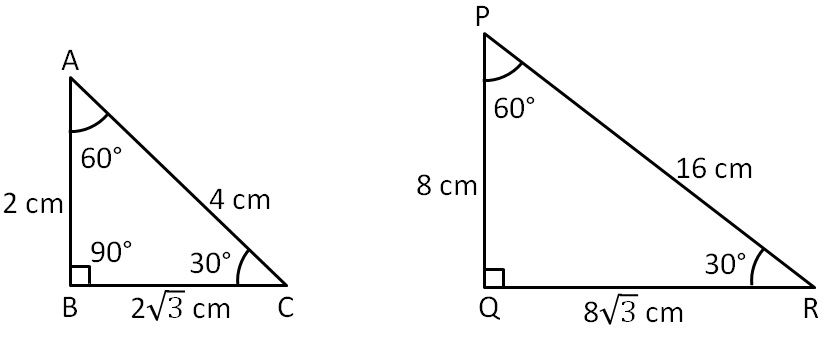Congruency Criteria Mix questions

Congruence of Triangles
Concept wise

Suppose we have two triangles,Here,

All the three angles of both triangle are equal,

But, sides are not equal

So, triangles are not congruent

i.e. ∆ABC ≇ ∆PQR

But,

These triangles are similar

Check AAA similarity criteria .

Learn in your speed, with individual attention - Teachoo Maths 1-on-1 Class